Hypercube, Tesseract
 What is the Hypercube? Cubes in Perspective Central Projections Nets Cross-Sections More Drawings The n-dimensional Cube The Hypercube on the Internet References .
 To the Main Page  "Mathematische Basteleien"

What is the Hypercube?
The hypercube is the cube with four dimensions.

Our imagination is not sufficient enough to understand the fourth dimension and the hypercube.
You can approach the hypercube through analogy to the 3-dimensional cube from different sides. So you can become familiar with it.

Cubes in Perspective top
 ...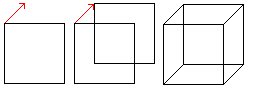... If you move a square parallel in plane and join the corresponding corners, you get the perspective sight of the cube.

 ...... If you move a cube parallel in space and join the corresponding corners, you get the perspective sight of the hypercube.
The hypercube has 16 corners (derived from 2 cubes) and 32 edges (2 cubes and joining lines).

 ...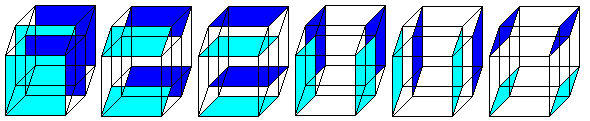...
The hypercube has 24 squares.

 ...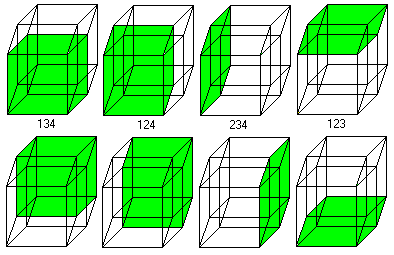... The cube is covered by six squares. In the same way eight cubes form the hypercube.
The numbers 134, 124, 234, 123 indicate the base vectors (declared below).

If you know the 3D view, you can look at the hypercube three-dimensionally, too.Central Projections top
 ...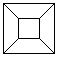......... The cube is distorted in a central projection. 4 of the 6 squares appear as trapeziums, which lie between the small and the big square.

 ....... A representation of the hypercube has been developed of this.  (Viktor Schlegel, 1888)

 ...... 6 of the 8 cubes appear as pyramid stumps,  which lie between the small and the big cube.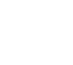4 cubes, 6 squares, and 4 edges meet at each corner.
3 cubes and 3 squares meet at each edge.
2 cubes meet at each square.

Nets    top
 ............ If you spread out the cube, you get its net. Together the six squares have 6x4=24 sides. 2x5=10 sides (red) are bound. If you build a cube, you have to stick the remaining 14 sides in pairs.  There are 11 nets.......... If you spread out the hypercube, you get its net as an arrangement of 8 cubes. Together the eight cubes have 8x6=48 squares. 2x7=14 squares are bound. If you "build" a hypercube, you have to stick the remaining 34 squares in pairs.  How many nets are there?  Peter Turney and Dan Hoey counted 261 cases.

Cross-Sections  top
 ..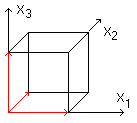... A cube (more exact: a cube with the edges 1) is produced by three unit vectors (red) perpendicular to each other. They form a coordinate system.

 ........... A triplet formed by the numbers 0 or 1 describes the corners. The triplet (011) belongs to the point P. You reach P by going from the origin O first in x2 direction and then in x3 direction. This way is fixed by 011.

 ...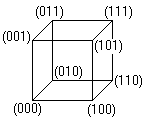...... You describe all the 8 corners by coordinates in this manner. All combinations of three numbers using 0 or 1 occur as coordinates.

 ............... If you add the coordinates of one point, you get the sums 0,1,2, or 3. The sums 0 and 3 belong to opposite corners. They are ending points of a diagonal (green). If you join the points with the sums 1 or 2, you get triangles (red).
If you write x1+x2+x3=a and substitute all numbers between 0 and 3 with a, you find to every value another plane. The hexagon corresponding to a=1.5 is famous.

top
 ...... Corresponding to the cube four basic vectors (red) produce the hypercube. All combinations of four numbers using 0 or 1 occur as coordinates.

 ......... If you add the coordinates of one point, you get the sums 0,1,2,3, or 4. The sums 0 and 4 belong to opposite corners. They are ending points of a diagonal (green). If you join the points with the sums 1 or 3, you get two tetrahedra (red). If you join the points with the sum 2, you get an octohedron (blue). If you write x1+x2+x3+x4=a and substitute all numbers between 0 and 4 with a, you find another body as a section to every value.  The section is perpendicular to the diagonal from (0000) to (1111).

More Drawings  top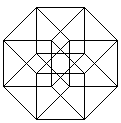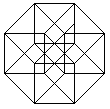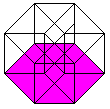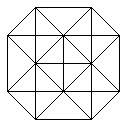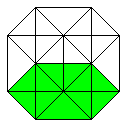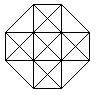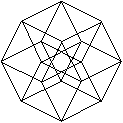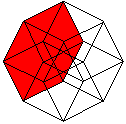The n-dimensional Cube   top
The hypercube is a construct of ideas. You receive a plausible explanation for its features by the "permanent principle", which often is used in mathematics to get from the "known to the unknown".
Cubes with the dimensions 1, 2 and 3 have the properties as follows.The data of the hypercube might follow in the next line. Dimension=4 and corners=16 are clear.
There are formulas for continuing the sequence for the edges and squares.If you take n=4, you get the data of the hypercube.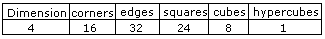Encore: Data of the 5-dimensional cube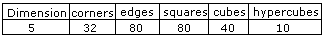The Hypercube on the Internet   top

English

Eric W. Weisstein, (MathWorld)
Hypercube

Peter Turney
Unfolding the Tesseract

Wikipedia
Hypercube, Tesseract, Fourth dimensionJoseph Cross (tower)

German

Frank Richter
living in the hyperspace

Hans Walser
Der n-dimensionale Hyperwürfel   (.pdf-Datei)

Manfred Hörsch
Hyperkubus - Videos

Roberto Neumann
Hyperwürfel und Hyperkugeln

Wikipedia
Hyperwürfel, Tesserakt, 4D, Josephskreuz

References  top
(1) Martin Gardner: Mathematischer Karneval, Frankfurt am Main, 1975
(2) R.Thiele, K.Haase: Der verzauberte Raum, Leipzig, 1991

Feedback: Email address on my main page